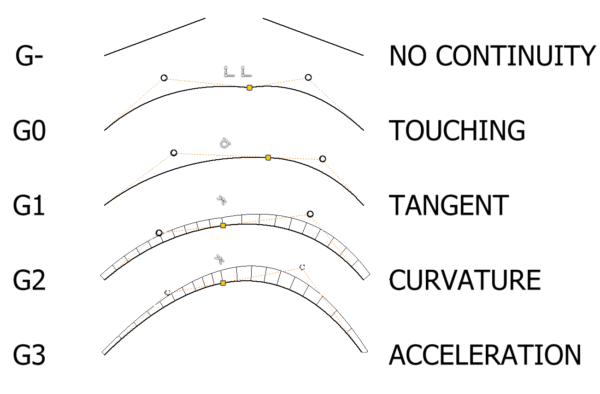# Autodesk Inventor surfacing. Curvature continuity and sketch constraints.

## Previously on CSO, we discussed the definition of Curvature continuity. In this post we will begin to discover how to create curvature continuous surfaces in Autodesk Inventor.

Let’s review our curvature definitions:

So – how are these definitions applied within a 2D sketch?### G0 = Coincident

G0 simply means that the two curves are touching. So a Coincident constraint will hold the endpoints of two curves in a G0 relationship (I’m using ‘Curves’ here as a catchall term for straight lines, arcs and splines).

### G1 = Coincident + Tangency

G1 adds tangency to the mix. You guessed it, applying a tangent constraint will hold our two curves in a G1 relationship.

### G2 = Coincident + Smooth

Inventor has a sketch constraint called ‘Smooth’. Applying this constraint will create a G2 relationship between our two curves, that is the two curves will have the same  curvature at the point where they they meet.

(you will find that you don’t need a Tangent constraint and a Smooth consistent, you can use use one or the other).

### G3 = ?

Unfortunately, Inventor doesn’t have a G3 constraint :/

However you can coax a G3 relationship out of a pair of curves by manually tweaking the curves until they look about right. The trick to this is to turn on curvature display by right clicking on the curve.

You can now compare the curvature of the two curves where they meet.If the curvature comb outlines are tangent to each other – congratulations, you’ve achieved G3. Well done you!